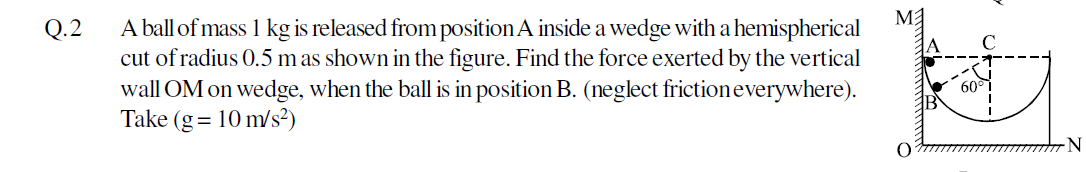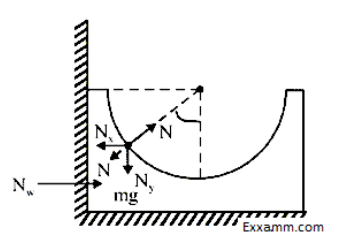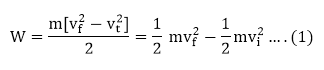# A ball of mass 1 kg is released from position A inside a wedge with a hemisphericalcut of radius 0.5 m as shown in the figure. Find the force exerted by the verticalwall OM on wedge, when the ball is in position B4 years agoThe Correct Answer is → 4
Applying WE theorem,
Wg + WN = ?KE
Mg (Rcos60°) + 0 = ½ mv2 – 0 ……………(i)
N – mg cos 60° = mv2/R ……………………(ii)
Nz – N sin 60° = Nw ……….. (iii)
By solving above equations
We get Nw = 15√3/2 N.
Relevant Theory, Formulas & Tricks
Work – Energy Theorem:
If a constant force F acts through a displacement x, it does work W = Fx = (ma)x on the particle.
Since the acceleration is constant, we can use the equation of kinematics
Thus,The quantity, x = ½ mv2 is a scalar and is called the kinetic energy of the participle. Kinetic energy is the energy that a particle posses by virtue of its motion. Thus, equation (1) takes the form
?U = - ?K ……………(2)
The work done by a force changes the kinetic energy of the particle. This is called the work Energy Theorem.
In general the net work done by the resultant of all the force acting on the particle is equal to the change in kinetic energy of a particle.
Wnet = ?k ………………(3)
And taking  into account the work done by internal forces, we have
Winternal + Wexternal = ?k
Important:
1.The kinetic energy of an object is a measure of the amount of work needed to increase its speed from zero to a given value.
2.The kinetic energy of a particle is the work it can do its surrounding in coming to rest.
3.Since the velocity and displacement of a particle depend on the frame of reference. The numerical values of the work and kinetic energy also depend on the frame.